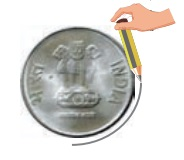Home | | Maths 4th Std | Draw circles using objects like bangles, coins etc

# Draw circles using objects like bangles, coins etc

Place a bangle or coin on a paper. Trace along its boundary till your Pencil reaches the starting point. This is a circle.

Draw circles using objects like bangles, coins etc.Place a bangle or coin on a paper. Trace along its boundary till your Pencil reaches the starting point. This is a circle.Activity

Let us draw the circle using a pencil and thread.

1. Mark a point A on a sheet.

2. Fix one end of the thread on the point and put the pencil on the other end of the thread as shown in the figure.

3. Move the pencil by keeping the thread at A. Point A is called the center of the circle.Tags : Geometry | Term 1 Chapter 1 | 4th Maths , 4th Maths : Term 1 Unit 1 : Geometry
Study Material, Lecturing Notes, Assignment, Reference, Wiki description explanation, brief detail
4th Maths : Term 1 Unit 1 : Geometry : Draw circles using objects like bangles, coins etc | Geometry | Term 1 Chapter 1 | 4th Maths